# 三角(反三角)函数在javascript中的应用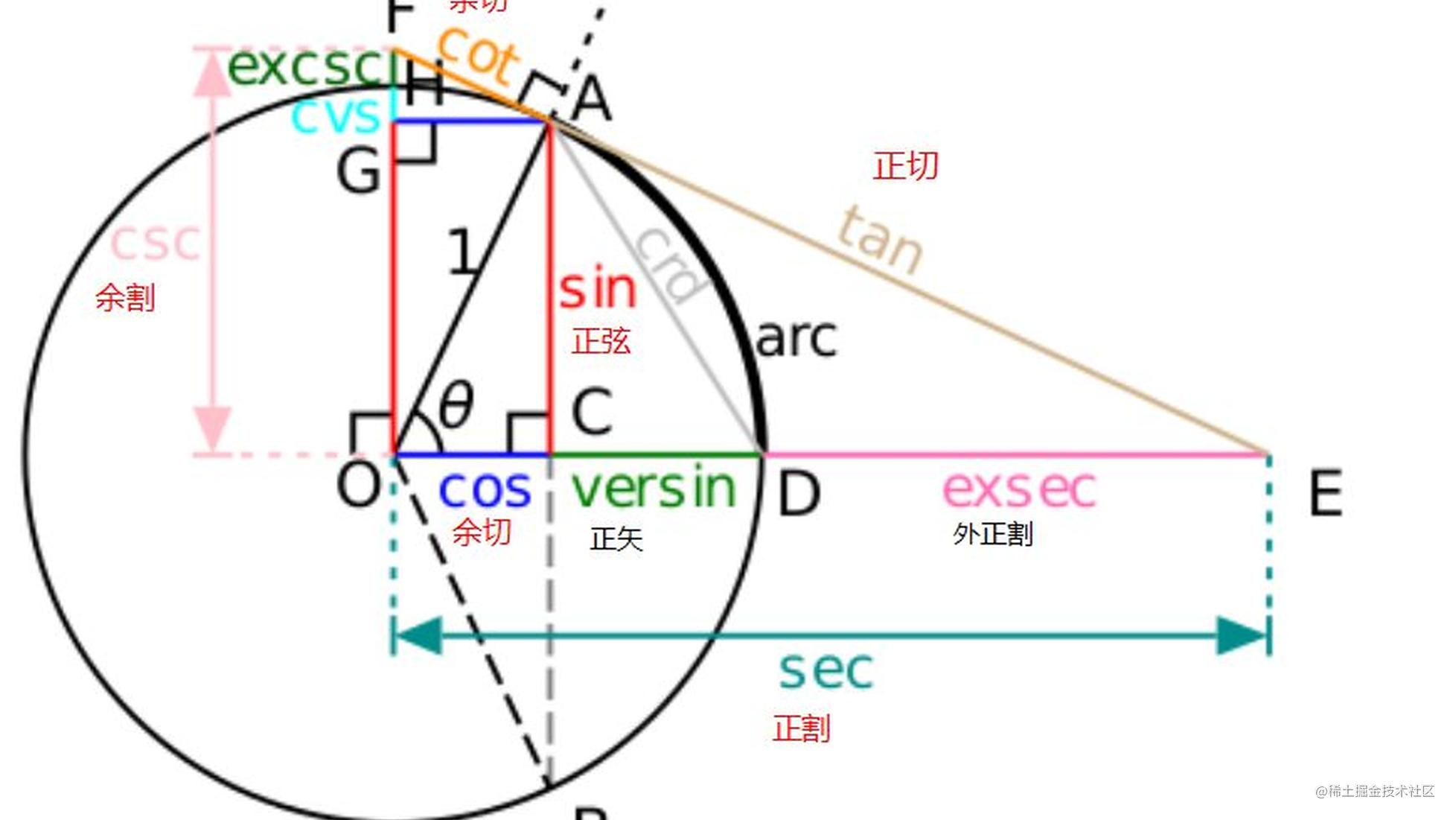# 三角函数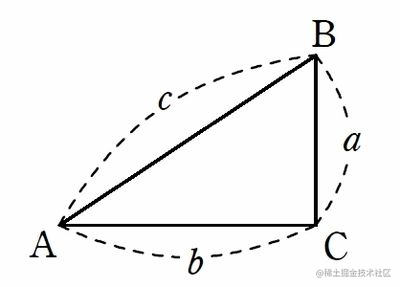Math.pow() 平方/立方

• Math.pow(2,3) // 2的立方
• Math.pow(2,2) // 2的平方

Math.sqrt() 开方

Math.sqrt(9) // 3 只能开平方，不能开立方或者是n次方

``````Math.sqrt(Math.pow(3,2)+Math.pow(4,2))  //5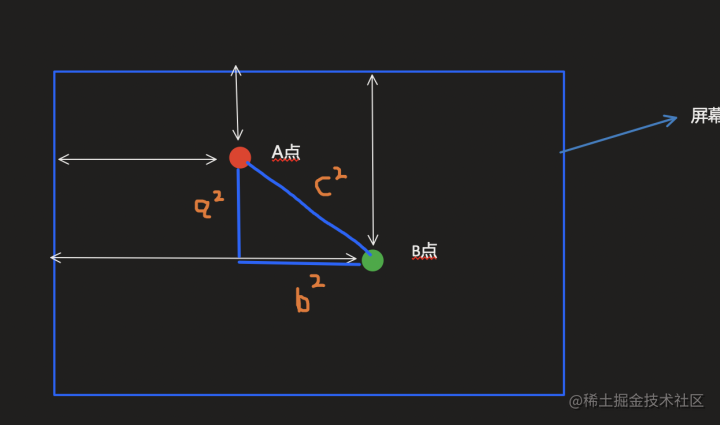# 三角函数

• sina
• cosa
• tana

• Math.sin(弧度)
• Math.cos(弧度)
• Math.tan(弧度)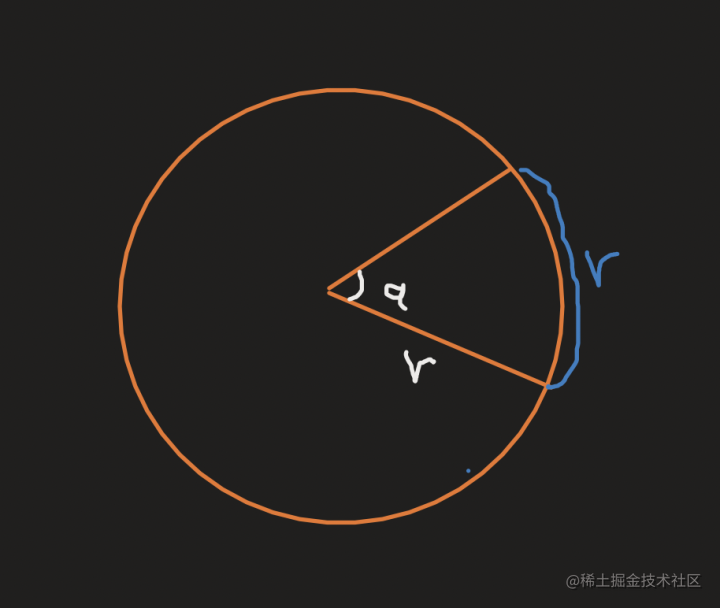π=3.1415926......

360度=2π(弧度)

``````Math.PI/180

``````Math.sin(30*Math.PI/180)   //30度角所对应的弧度==>0.5

π在javascript中是一个常量 用Math.PI表示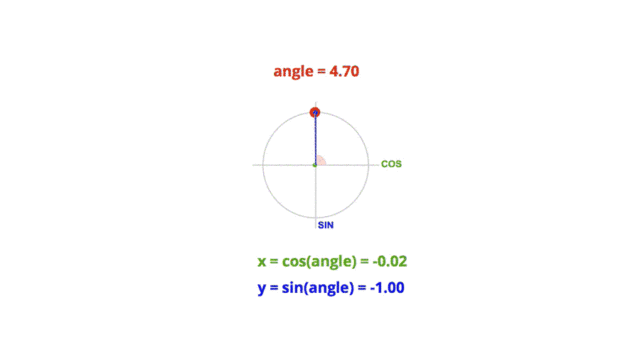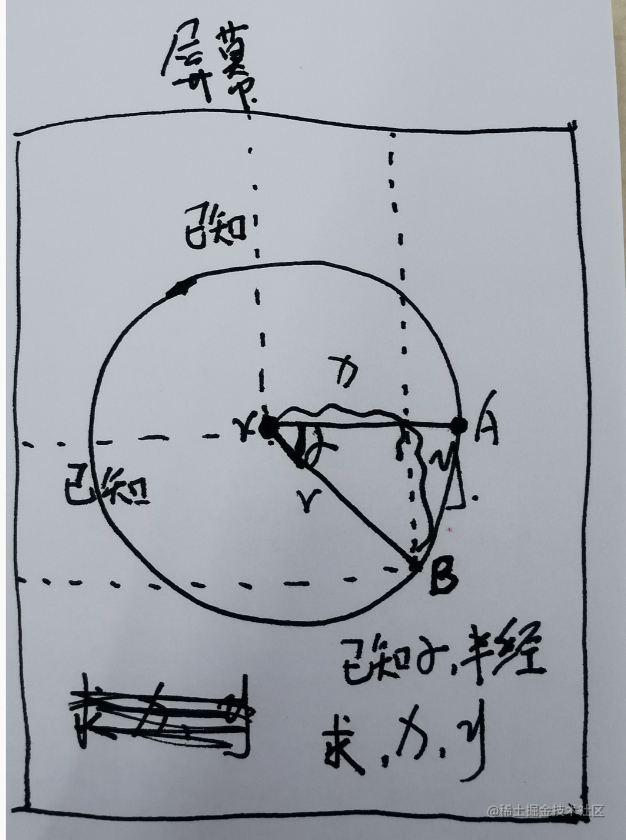``````Math.sin( 度数*Math.PI/180 ) = y/r
Math.cos( 度数*Math.PI/180 ) = x/r

github.com/wensiyuanse…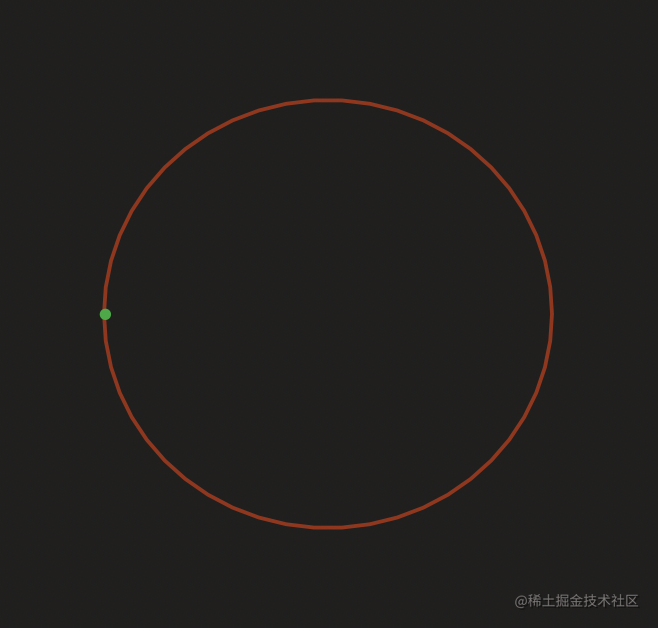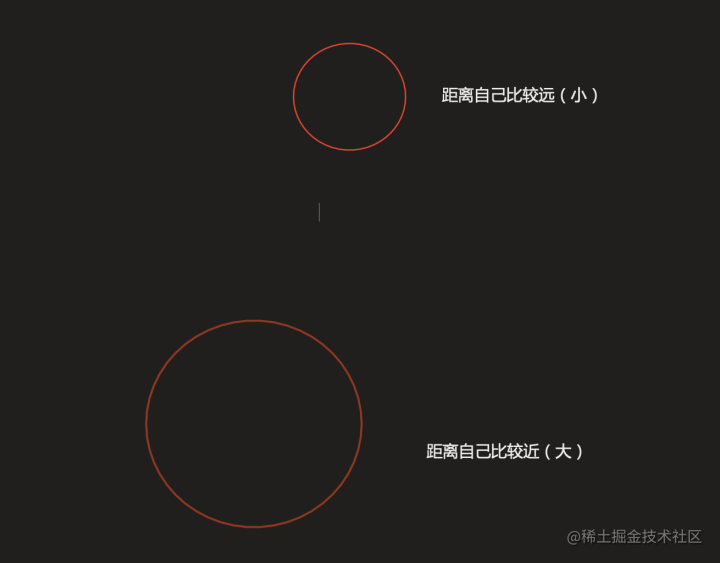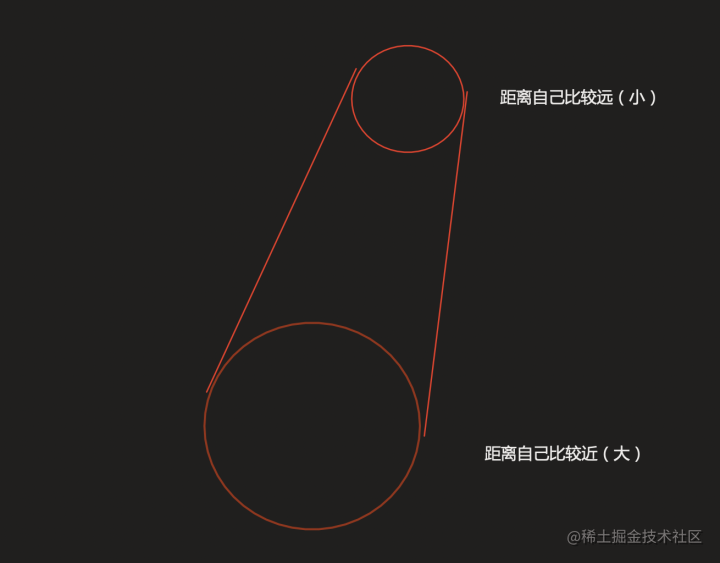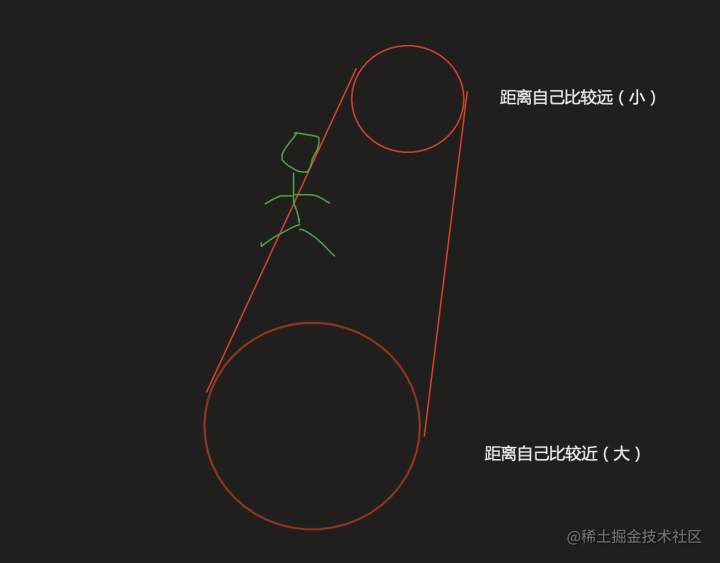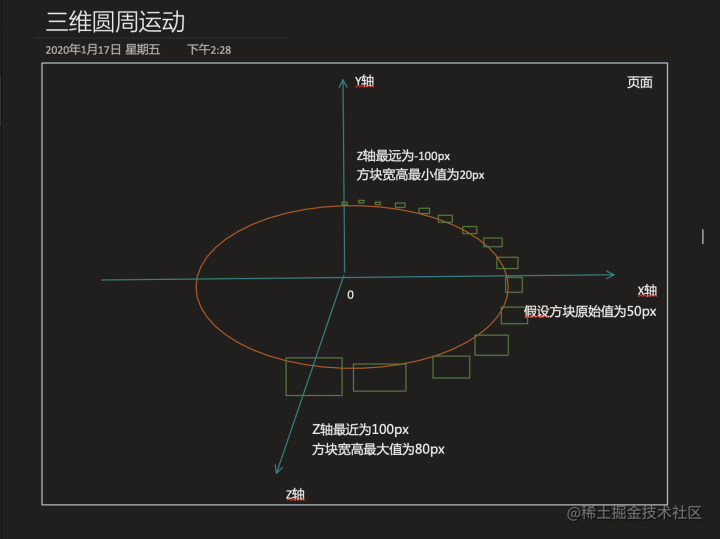• X轴控制物体的let值
• Y轴是不变===>元素的top值不变
• Z轴越大说明物体就越大，Z轴越小说明物体就越小

``````var x = 700;
var a = Math.sin(度数 * Math.PI / 180) * r;
var b = Math.cos(度数 * Math.PI / 180) * r;
oDiv.style.left = x + b + 'px';
oDiv.style.width = a / 100 * 30 + 50 + 'px'; //100为半径，30为比率，50是方块的原始宽高
oDiv.style.height = a / 100 * 30 + 50 + 'px';

# 反三角函数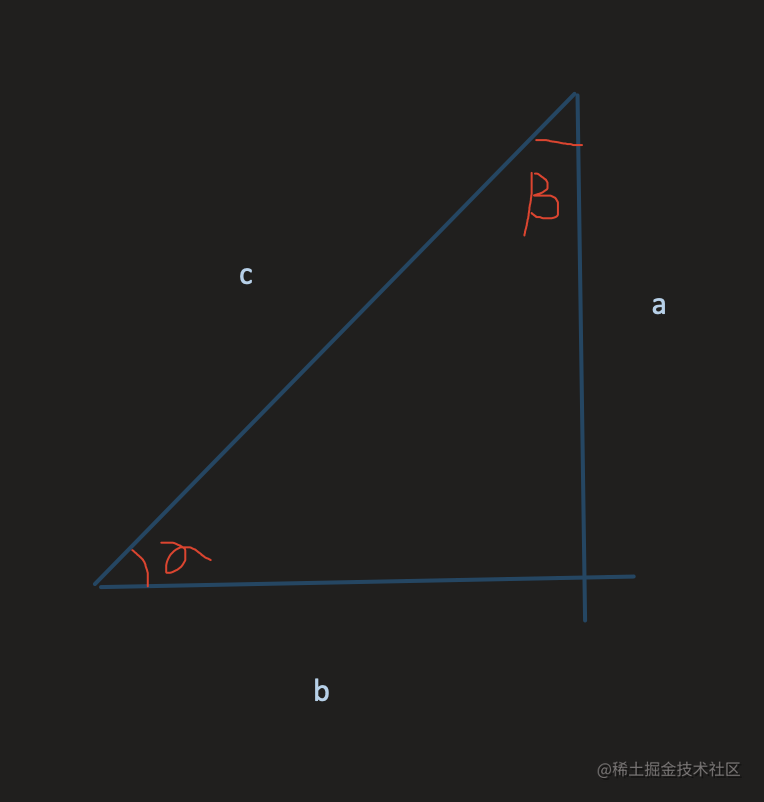• Math.asin( a/c )
• Math.acos( b/c )
• Math.atan( a/b )

``````Math.asin(1/2)  //0.5弧度

``````Math.asin(1/2)*180/Math.PI  //30度

# 三角函数图像

juejin.cn/post/684490…

## 单位圆坐标系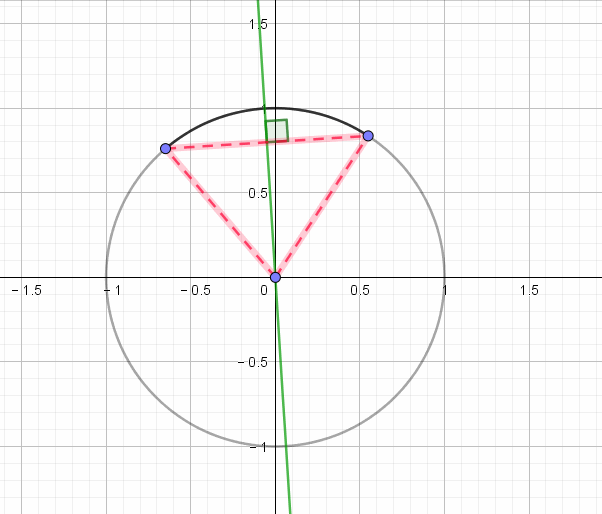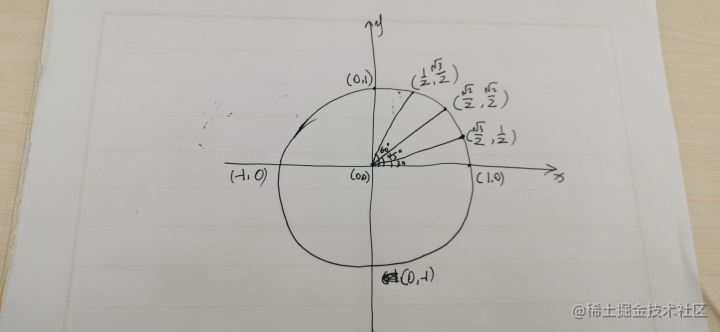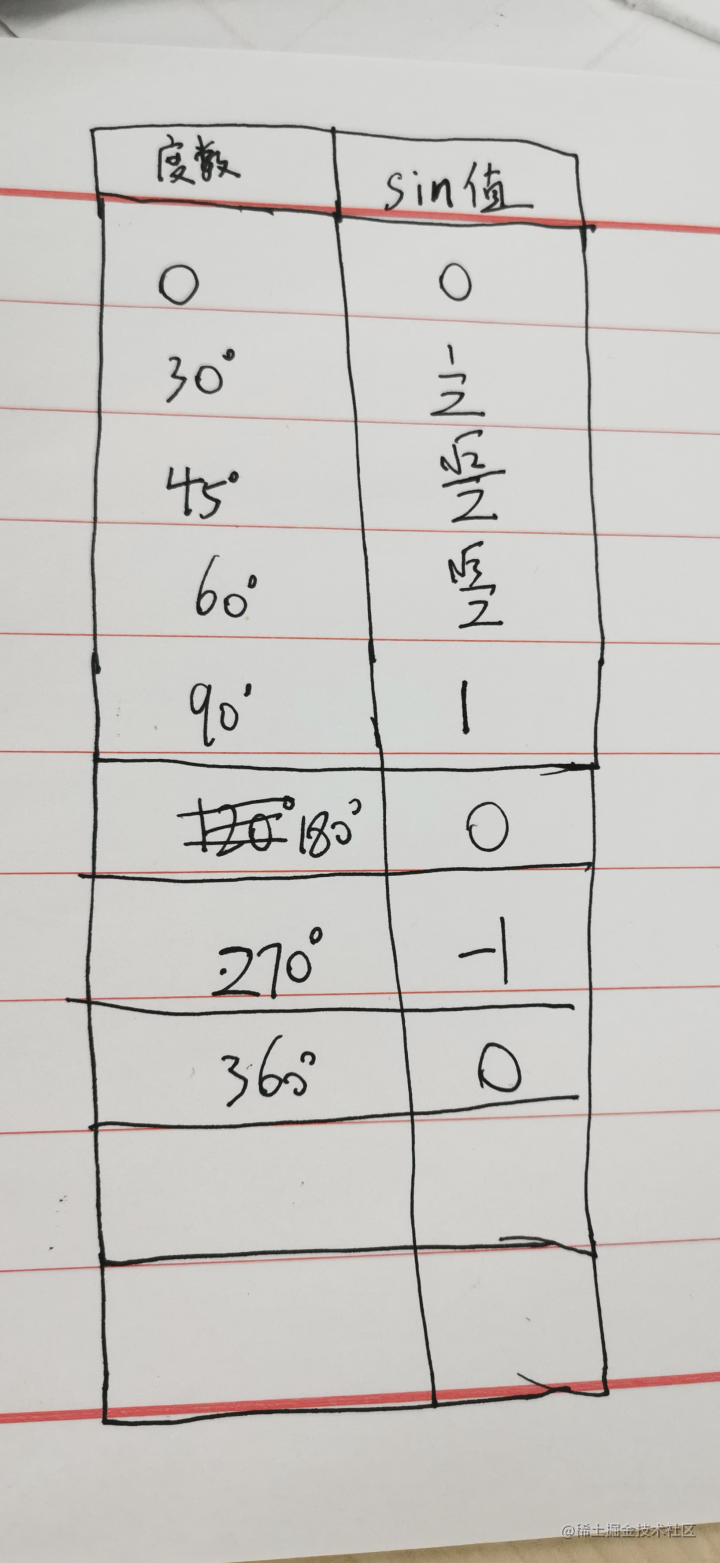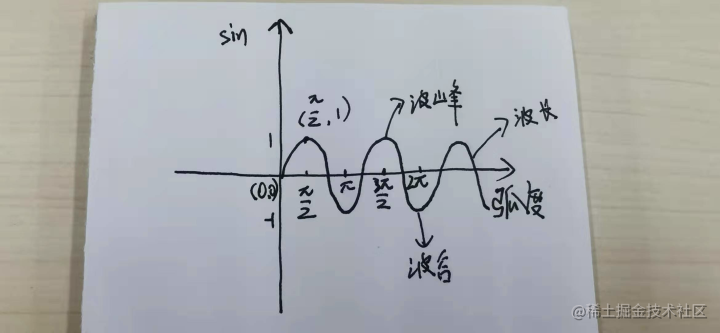github.com/wensiyuanse…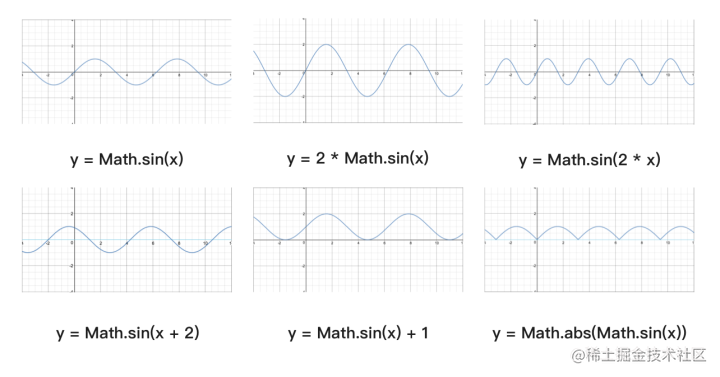• A 控制振幅，A 值越大，波峰和波谷越大，A 值越小，波峰和波谷越小；

• B 值会影响周期，B 值越大，那么周期越短，B 值越小，周期越长。

• C 值会影响图像左右移动，C 值为正数，图像右移，C 值为负数，图像左移。

• D 值控制上下移动。

www.w3cplus.com/javascript/…